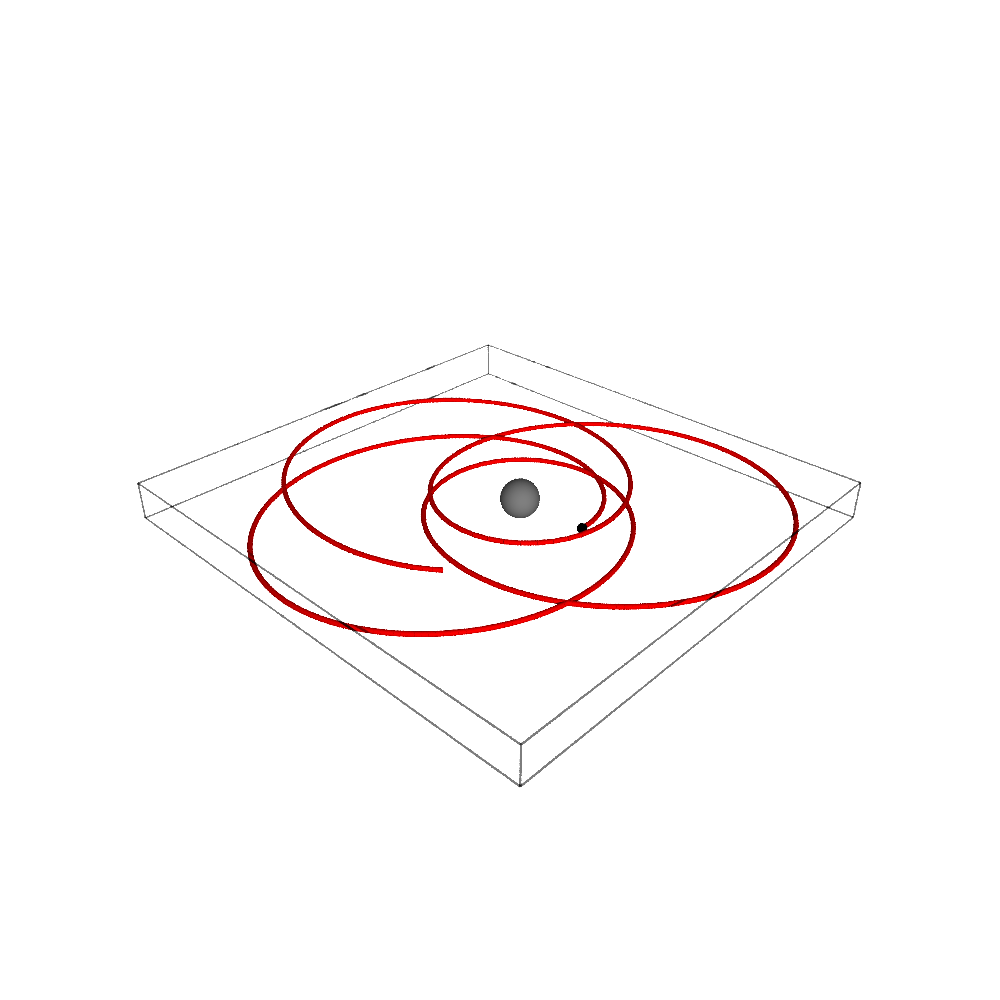# Timelike geodesic in Schwarzschild spacetime¶

This Jupyter/SageMath notebook presents the numerical computation of a timelike geodesic in Schwarzschild spacetime, given an initial point and tangent vector. It uses the integrated_geodesic functionality introduced by Karim Van Aelst in SageMath 8.1, in the framework of the SageManifolds project.

Click here to download the notebook file (ipynb format). To run it, you must start SageMath within the Jupyter notebook, via the command sage -n jupyter

A version of SageMath at least equal to 8.2 is required to run this notebook:

In :
version()

Out:
'SageMath version 8.3, Release Date: 2018-08-03'
In :
%display latex  # LaTeX rendering turned on


We define first the spacetime manifold $M$ and the standard Schwarzschild-Droste coordinates on it:

In :
M = Manifold(4, 'M', structure='Lorentzian')
X.<t,r,th,ph> = M.chart(r't r:(0,+oo) th:(0,pi):\theta ph:\phi')
X

Out:

For graphical purposes, we introduce $\mathbb{R}^3$ and some coordinate map $M\to \mathbb{R}^3$:

In :
R3 = Manifold(3, 'R^3', latex_name=r'\mathbb{R}^3')
X3.<x,y,z> = R3.chart()
to_R3 = M.diff_map(R3, {(X, X3): [r*sin(th)*cos(ph),
r*sin(th)*sin(ph), r*cos(th)]})
to_R3.display()

Out:

Then, we define the Schwarzschild metric:

In :
g = M.metric()
m = var('m'); assume(m >= 0)
g[0,0], g[1,1] = -(1-2*m/r), 1/(1-2*m/r)
g[2,2], g[3,3] = r^2, (r*sin(th))^2
g.display()

Out:

We pick an initial point and an initial tangent vector:

In :
p0 = M.point((0, 8*m, pi/2, 1e-12), name='p_0')
v0 = M.tangent_space(p0)((1.297513, 0, 0, 0.0640625/m), name='v_0')
v0.display()

Out:

We declare a geodesic with such initial conditions, denoting by $s$ the affine parameter (proper time), with $(s_{\rm min}, s_{\rm max})=(0, 1500\,m)$:

In :
s = var('s')
geod = M.integrated_geodesic(g, (s, 0, 1500), v0); geod

Out:

We ask for the numerical integration of the geodesic, providing some numerical value for the parameter $m$, and then plot it in terms of the Cartesian chart X3 of $\mathbb{R}^3$:

In :
sol = geod.solve(parameters_values={m: 1})  # numerical integration
interp = geod.interpolate()                 # interpolation of the solution for the plot
graph = geod.plot_integrated(chart=X3, mapping=to_R3, plot_points=500,
thickness=2, label_axes=False)           # the geodesic
graph += p0.plot(chart=X3, mapping=to_R3, size=4, parameters={m: 1}) # the starting point
graph += sphere(size=2, color='grey')                                 # the event horizon
show(graph, viewer='threejs', online=True)


A Tachyon view of the geodesic:

In :
show(graph, viewer='tachyon', aspect_ratio=1, figsize=10)Some details about the system solved to get the geodesic:

In :
geod.system(verbose=True)

Geodesic in the 4-dimensional Lorentzian manifold M equipped with Lorentzian metric g on the 4-dimensional Lorentzian manifold M, and integrated over the Real interval (0, 1500) as a solution to the following geodesic equations, written with respect to Chart (M, (t, r, th, ph)):

Initial point: Point p_0 on the 4-dimensional Lorentzian manifold M with coordinates [0, 8*m, 1/2*pi, 1.00000000000000e-12] with respect to Chart (M, (t, r, th, ph))
Initial tangent vector: Tangent vector v_0 at Point p_0 on the 4-dimensional Lorentzian manifold M with components [1.29751300000000, 0, 0, 0.0640625000000000/m] with respect to Chart (M, (t, r, th, ph))

d(t)/ds = Dt
d(r)/ds = Dr
d(th)/ds = Dth
d(ph)/ds = Dph
d(Dt)/ds = 2*Dr*Dt*m/(2*m*r - r^2)
d(Dr)/ds = -(4*Dth^2*m^2*r^3 - 4*Dth^2*m*r^4 + Dth^2*r^5 - 4*Dt^2*m^3 + 4*Dt^2*m^2*r + (Dr^2 - Dt^2)*m*r^2 + (4*Dph^2*m^2*r^3 - 4*Dph^2*m*r^4 + Dph^2*r^5)*sin(th)^2)/(2*m*r^3 - r^4)
d(Dth)/ds = (Dph^2*r*cos(th)*sin(th) - 2*Dr*Dth)/r
d(Dph)/ds = -2*(Dph*Dth*r*cos(th) + Dph*Dr*sin(th))/(r*sin(th))


Out:

We recognize in the above list the Christoffel symbols of the metric $g$:

In :
g.christoffel_symbols_display()

Out:
In :
g.riemann().display_comp()

Out:
In [ ]: